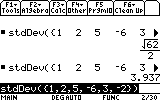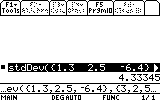# Knowledge Base

## Solution 11712: Calculating Standard Deviation on the TI-89 Family, TI-92 Family, and Voyage™ 200 Graphing Calculators.

### How do I calculate standard deviation on my TI-89 family, TI-92 family, or Voyage 200 graphing calculator?

Standard deviation can be calculated using several methods on the TI-89 family, TI-92 family and Voyage 200 graphing calculators. Standard deviation can be calculated by using the stdDev() function. The stdDev() function can be located by performing the following:

• Press [2ND] [MATH]
• Scroll to 6:Statistics and select 6:stdDev(

Follow the examples listed below to calculate standard deviation of one or two list of data.

Ex. Calculate the Standard Deviation of {1, 2, 5, -6, 3, -2}

• From the HOME screen, press [2ND] [MATH]
• Select 6:Statistics and 6:stdDev
• Input the data {1, 2, 5, -6, 3, -2}, and press [)]
• Once the screen displays stdDev({1, 2, 5, -6, 3, -2}) press [ENTER] to calculate the result of 3.937 or square-root (62)/2Ex. Calculate the Standard Deviation of {1.3, 2.5, -6.4} and {3, 2, 5}

• From the HOME screen, press [2ND] [MATH]
• Select 6:Statistics and 6:stdDev
• Input the data {1.3, 2.5, -6.4} , {3, 2, 5} and press [)]
• Once the screen displays stdDev({1.3, 2.5, -6.4} , {3, 2, 5}) press [ENTER] to calculate the result of 4.33345The standard deviation is also calculated and displayed when 1-variable or 2-variable statistics are calculated.

Please see the TI-89 family, TI-92 family, and Voyage 200 guidebooks for additional information.